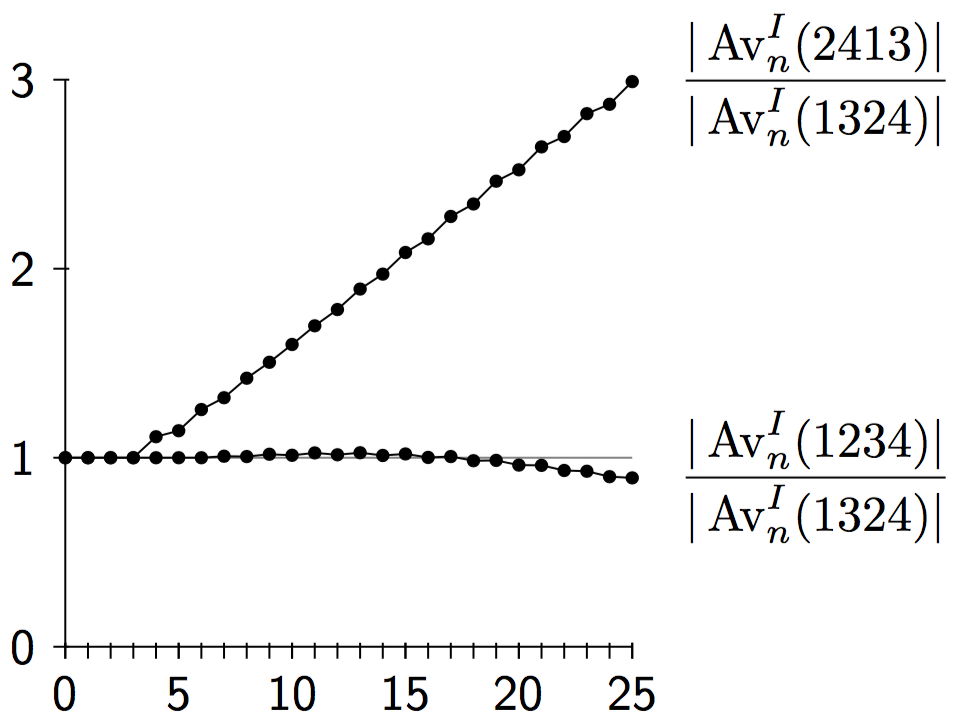# Jay Pantone

## Pattern-Avoiding Involutions: Exact and Asymptotic Enumeration

We consider the enumeration of pattern-avoiding involutions, focusing in particular on sets defined by avoiding a single pattern of length 4. We directly enumerate the involutions avoiding $$1342$$ and the involutions avoiding $$2341$$. As we demonstrate, the numerical data for these problems exhibits some surprising behavior. This strange behavior even provides some very unexpected data related to the number of $$1324$$-avoiding permutations.

##### Acknowledgments

We are very grateful to Michael Albert for adding support for involutions to his PermLab package.

##### OEIS Sequences
• A230551 - $$\operatorname{Av}^I(2431)$$
• A230552 - $$\operatorname{Av}^I(2341)$$
• A230553 - $$\operatorname{Av}^I(1342)$$
• A001006 - $$\operatorname{Av}^I(1234)$$
• A230554 - $$\operatorname{Av}^I(1324)$$
• A230555 - $$\operatorname{Av}^I(3421)$$
• A230556 - $$\operatorname{Av}^I(4231)$$
• A121704 - $$\operatorname{Av}^I(2413)$$
##### Open Questions
• The enumerations (and, in particular, the exponential growth rates) of $$\operatorname{Av}^I(2431)$$, $$\operatorname{Av}^I(1324)$$, $$\operatorname{Av}^I(3421)$$, and $$\operatorname{Av}^I(4231)$$ are still unknown.
• The growth rate of the sequence $$|\text{Av}_n^I(2413)|$$ is $$\approx 3.15$$, while the growth rate of $$|\text{Av}_n^I(1324)|$$ is known to be at least 3.13 and forthcoming results imply it is at least 3.20. Therefore, the ratios $$\displaystyle \frac{|\text{Av}_n^I(2413)|}{|\text{Av}_n^I(1324)|}$$ eventually approach zero. However, as the chart below shows, the plot of the first 25 ratios is steadily increasing. When does the plot begin to decrease? When does it fall below 1? Understanding this may help understand the sequence $$|\text{Av}_n(1324)|$$.